# Linear Impulse Momentum Relations (Part -1) Civil Engineering (CE) Notes | EduRev

## Civil Engineering (CE) : Linear Impulse Momentum Relations (Part -1) Civil Engineering (CE) Notes | EduRev

The document Linear Impulse Momentum Relations (Part -1) Civil Engineering (CE) Notes | EduRev is a part of the Civil Engineering (CE) Course Introduction to Dynamics and Vibrations- Notes, Videos, MCQs.
All you need of Civil Engineering (CE) at this link: Civil Engineering (CE)

4.2 Linear impulse-momentum relations

In most dynamic problems, particles are subjected to forces that vary with time. We can write this mathematically by saying that the force is a vector valued function of time F(t) . If we express the force as components in a fixed basis {i,j,k} then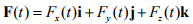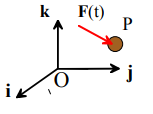where each component of the force is a function of time.

The Linear Impulse exerted by a force during a time interval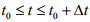is defined as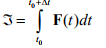The linear impulse is a vector, and can be expressed as components in a basis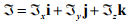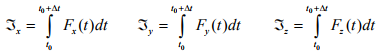If you know the force as a function of time, you can calculate its impulse using simple calculus. For example:

1. For a constant force, with vector value F0, the impulse is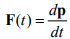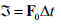2. For a harmonic force of the form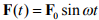the impulse is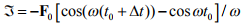It is rather rare in practice to have to calculate the impulse of a force from its time variation.

4.2.2 Definition of the linear momentum of a particle

The linear momentum of a particle is simply the product of its mass and velocity

P = mv

The linear momentum is a vector – its direction is parallel to the velocity of the particle.

4.2.3 Impulse-momentum relations for a single particle

Consider a particle that is subjected to a force F(t) for a time interval.
Let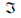denote the impulse exerted by F on the particle
Let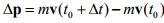denote the change in linear momentum during the time interval Δt.

The momentum conservation equation can be expressed either in differential or integral form.

1. In differential formThis is clearly just a different way of writing Newton’s law F=ma.

2. In integral form= Δp

This is the integral of Newton’s law of motion with respect to time.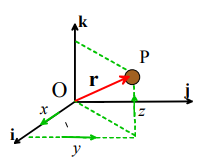The impulse-momentum relations for a single particle are useful if you need to calculate the change in velocity of an object that is subjected to a prescribed force.

4.2.4 Examples using impulse-momentum relations for a single particle

Example 1: Estimate the time that it takes for a Ferrari Testarossa traveling at the RI speed limit to make an emergency stop. (Like many textbook problems this one is totally unrealistic – nobody in a Ferrari is going to travel at the speed limit!)We can do this calculation using the impulse-momentum relation for a single particle. Assume that the car has mass m, and travels with speed V before the brakes are applied. Let Δt denote the time required to stop.

1. Start by estimating the force acting on the car during an emergency stop. The figure shows a free body diagram for the car as it brakes to a standstill. We assume that the driver brakes hard enough to lock the wheels, so that the car skids over the road. The horizontal friction forces must oppose the sliding, as shown in the picture. F=ma for the car follows as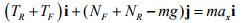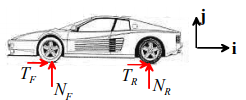The vertical component of the equation of motion shows that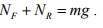The friction law then shows that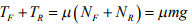2. The force acting on the car is constant, so the impulse that brings the car to a halt is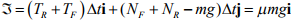3. The linear momentum of the car before the brakes are applied is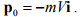The linear momentum after the car stops is zero. Therefore,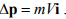4. The linear impulse-momentum relation shows that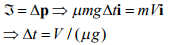5. We can take the friction coefficient as μ ≈ 0.8 , and 65mph is 29m/s. We take the gravitational acceleration g = 9.81 . The time follows as Δt ≈ 3.7s . Note that a testarossa cant stop any faster than a Honda Civic, despite the price difference.....

Example 2: The figure shows a pendulum that swings with frequency f swings per second. Find an expression for the average reaction force at the pivot of a pendulum during one cycle of oscillation. (This seems a totally academic problem – and indeed it is – but the important point is that certain quantities, such as average forces, can often be computed without solving the full equations of motion. These quantities can be extremely useful for checking computer simulations)Calculation: Consider the pendulum at the extreme limits of its swing, at the start and end of one cycle of oscillation. Note that

1. The velocity of the particle is zero both at the start and end of the cycle. Its change in momentum is therefore zero, and the total impulse exerted on the particle must be zero.

2. The particle is subjected to two forces (i) gravity; and (ii) the reaction force exerted on the particle. During a swing of the pendulum these forces exert impulses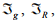respectively.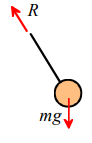3. The impulse exerted by the gravitational force is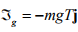where T = 1 / f is the time for one complete swing of the pendulum.

4. Since the total impulse is zero, it follows that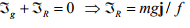The MATLAB script below shows how this result can be used to check the program developed in Section ???? to model the swing of a pendulum.

function pendulum_reactions

g = 9.81;
l = 1;
m = 1;
theta = pi*45/180;
time = 10;
Abstol = 10^(-03)*ones(4,1);
options = odeset('RelTol',0.000001,'AbsTol',Abstol,'Events',@event);
y0 = [l*sin(theta),l*cos(theta),0,0]; % Initial conditions
[t,y,tevent,yevent,ievent] = ode45(@eom,[0,time],y0,options);%,options);
plot(t,y(:,1:2));

% Calculate and plot the reaction forces as a function of time
for i=1:length(t)
[Reaction(i,1),Reaction(i,2)] = reactions(y(i,:));

end
figure
plot(t,Reaction(:,1:2));
% Integrate the reaction forces with respect to time
Ix = trapz(t,Reaction(:,1))
Iy = trapz(t,Reaction(:,2))

AverageRy = trapz(t,Reaction(:,2))/time(length(t))

function dydt = eom(t,y)
% The vector y contains [x,y,vx,vy]
M = eye(5); % This sets up a 5x5 matrix with 1s on all the diags
M(3,5) = (y(1)/m)/sqrt(y(1)^2+y(2)^2);
M(4,5) = (y(2)/m)/sqrt(y(1)^2+y(2)^2);

M(5,3) = y(1);
M(5,4) = y(2);
M(5,5) = 0;
f = [y(3);y(4);0;g;-y(3)^2-y(4)^2];
sol = M\f; % This solves the matrix equation M*[dydt,R]=f for [dydt,R]
dydt = sol(1:4); % only need to return time derivatives dy/dt
end

function [Rx,Ry] = reactions(y)
% This function calculates the reaction forces Rx, Ry, given the vector
% y containing [x,y,vx,vy]
M = eye(5); % This sets up a 5x5 matrix with 1s on all the diags
M(3,5) = (y(1)/m)/sqrt(y(1)^2+y(2)^2);
M(4,5) = (y(2)/m)/sqrt(y(1)^2+y(2)^2);
M(5,3) = y(1);
M(5,4) = y(2);
M(5,5) = 0;
f = [y(3);y(4);0;g;-y(3)^2-y(4)^2];
sol = M\f; % This solves the matrix equation M*[dydt,R]=f for [dydt,R]
Rx = -sol(5)*y(1)/sqrt(y(1)^2+y(2)^2);
Ry = -sol(5)*y(2)/sqrt(y(1)^2+y(2)^2);
end

function [eventvalue,stopthecalc,eventdirection] = event(t,y)
% Function to stop the calculation after one swing %
Note that v_x=0 at the end of the swing and crosses zero from above
eventvalue = y(3);
stopthecalc = 1; % This stops the calculation
eventdirection = -1;
end

end

4.2.5 Impulse-momentum relation for a system of particles

Suppose we are interested in calculating the motion of several particles, sketched in the figure.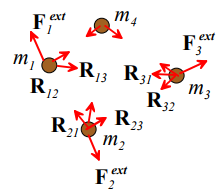Total external impulse on a system of particles: Each particle in the system can experience forces applied by:

• Other particles in the system (e.g. due to gravity, electric charges on the particles, or because the particles are physically connected through springs, or because the particles collide). We call these internal forces acting in the system. We will denote the internal force exerted by the ith particle on the jth particle by Rij.  Note that, because every action has an equal and opposite reaction, the force exerted on the jth particle by the ith particle must be equal and opposite, to Rij, i,e. Rij = - Rij.
• Forces exerted on the particles by the outside world (e.g. by externally applied gravitational or electromagnetic fields, or because the particles are connected to the outside world through mechanical linkages or springs). We call these external forces acting on the system, and we will denote the external force on the i th particle by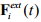We define the total impulse exerted on the system during a time interval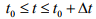as the sum of all the impulses on all the particles. It’s easy to see that the total impulse due to the internal forces is zero – because the ith and jth particles must exert equal and opposite impulses on one another, and when you add them up they cancel out. So the total impulse on the system is simply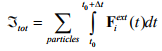Total linear momentum of a system of particles: The total linear momentum of a system of particles is simply the sum of the momenta of all the particles, i.e.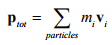The impulse-momentum equation can be expressed either in differential or integral form, just as for a single particle:

1. In differential form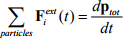This is clearly just a different way of writing Newton’s law F=ma

2. In integral form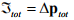This is the integral of Newton’s law of motion with respect to time.

Conservation of momentum: If no external forces act on a system of particles, their total linear momentum is conserved, i.e. Δp = 0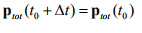4.2.6 Examples of applications of momentum conservation for systems of particles

The impulse-momentum equations for systems of particles are particularly useful for (i) analyzing the recoil of a gun; and (ii) analyzing rocket and jet propulsion systems. In both these applications, the internal forces acting between the gun on the projectile, or the motor and propellant, are much larger than any external forces, so the total momentum of the system is conserved.

Example 1: Estimate the recoil velocity of a rifle (youtube abounds with recoil demonstrations – see. e.g.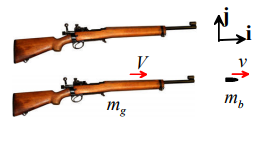The recoil velocity can be estimated by noting that the total momentum of bullet and rifle together must be conserved. If we can estimate the mass of rifle and bullet, and the bullet’s velocity, the recoil velocity can be computed from the momentum conservation equation.

Assumptions:

1. The mass of a typical 0.22 (i.e. 0.22” diameter) caliber rifle bullet is about 7 x 10- 4 kg (idealizing the bullet as a sphere, with density 7860 kg / m3)
2. The muzzle velocity of a 0.22 is about 1000 ft/sec (305 m/s)
3. A typical rifle weighs between 5 and 10 lb (2.5-5 kg)

Calculation:

1. Let mb denote the bullet mass, and let mR denote the mass of the rifle.
2. The rifle and bullet are idealized as two particles. Before firing, both are at rest. After firing, the bullet has velocity vb = vi ; the rifle has velocity vR = Vi .
3. External forces acting on the system can be neglected, so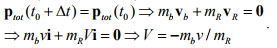4. Substituting numbers gives |V| between 0.04 and 0.08 m/s (about 0.14 ft/sec)

Example 2: Derive a formula that can be used to estimate the mass of a handgun required to keep its recoil within acceptable limits.

The preceding example shows that the firearm will recoil with a velocity that depends on the ratio of the mass of the bullet to the firearm. The firearm must be brought to rest by the person holding it.

Assumptions:

1. We will idealize a person’s hand holding the gun as a spring, with stiffness k, fixed at one end. The ‘end-point stiffness’ of a human hand has been extensively studied – see, e.g. Shadmehr et al J. Neuroscience, 13 (1) 45 (1993). Typical values of stiffness during quasi-static deflections are of order 0.2 N/mm. During dynamic loading stiffnesses are likely to be larger than this.
2. We idealize the handgun and bullet as particles, with mass mb and mg , respectively.

Calculation.

1. The preceding problem shows that the firearm will recoil with velocity V = -mbv / mg
2. Energy conservation can be used to calculate the recoil distance. We consider the firearm and the hand holding it a system. At time t=0 it has zero potential energy; and has kinetic energy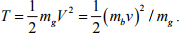At the end of the recoil, the gun is at rest, and the spring is fully compressed – the kinetic energy is zero, and potential energy is 1/2 kd2. Energy conservation gives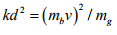3. The required mass follows as mg = (mbv)2 / kd2.

4. Substituting numbers gives

Offer running on EduRev: Apply code STAYHOME200 to get INR 200 off on our premium plan EduRev Infinity!

## Introduction to Dynamics and Vibrations- Notes, Videos, MCQs

20 videos|53 docs

,

,

,

,

,

,

,

,

,

,

,

,

,

,

,

,

,

,

,

,

,

;